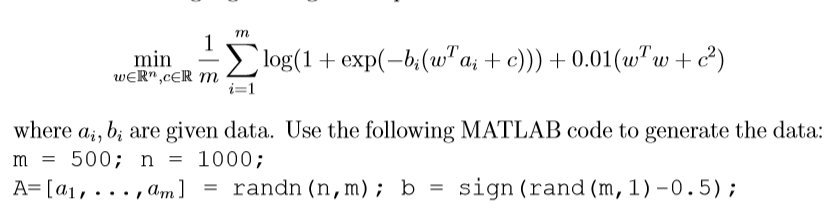# 请问逻辑回归的函数如何在MATLAB中求导以及求HESSIAN矩阵？``````function [ outcome ] = f( w,c,A,b )
n=size(A,1);
m=size(A,2);
outcome=0;
for i=1:m
outcome=outcome+log(1+exp(-b(i)*(w'*A(:,i)+c)));
end
outcome=(outcome+0.01*(w'*w+c*c))/m;
end
``````2019/05/03 14:28
• 机器学习
• 深度学习
• 开发语言
• 点赞
• 收藏
• 回答
私信